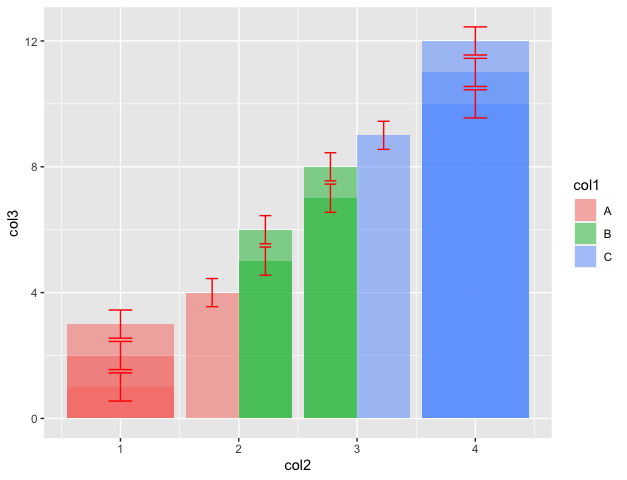# Grouped barplot in R with error bars

• Last Updated : 22 Nov, 2021

In this article, we are going to see how to create grouped barplot in the R programming language with error bars.

A data frame can be created in R working space using the data.frame() method. The tidyverse package is installed and loaded into the working space in order to perform data mutations and manipulations.

The package can be incorporated in the working space using the following command:

`install.packages("tidyverse")`

The declared data frame is subjected to a large number of operations using the pipe operator. Initially, the group_by method is applied to segregate data in different groups. It takes as argument the column to group data by.

Syntax: group_by (col-name)

Arguments :

• col-name – The column to group data by

A temporary column can then be added by performing the mathematical computation of dividing the values of standard deviation of the required column with its length. The standard deviation is calculated using the sd() method. The length can be computed by the length() method. Both these methods take as an argument the column names. The column can be appended to the data frame using the mutate() method.

`mutate (new-col-name = func())`

The ggplot method in R is used to do graph visualizations using the specified data frame. It is used to instantiate a ggplot object. Aesthetic mappings can be created to the plot object to determine the relationship between the x and y-axis respectively. Additional components can be added to the created ggplot object.

Syntax: ggplot(data = NULL, mapping = aes(), fill = )

Arguments :

• data – Default dataset to use for plot.
• mapping – List of aesthetic mappings to use for plot.

Geoms can be added to the plot using various methods. The geom_line() method in R can be used to add graphical lines in the plots made. It is added as a component to the existing plot. Aesthetic mappings can also contain color attributes which is assigned differently based on different data frames.

The geom_bar() method is used to construct the height of the bar proportional to the number of cases in each group.

Syntax: geom_bar ( width, stat)

Arguments :

• width – Bar width

The geom_errorbar() method is used to add error bars to the plot.

Syntax: geom_errorbar(mapping = NULL, data = NULL, stat = “identity”, position = “identity”, …)

Arguments :

• mapping – The aesthetic mapping, usually constructed with aes or aes_string.
• stat – The statistical transformation to use on the data for this layer.
• position – The position adjustment to use for overlapping points on this layer

## R

 `# importing the required library``library``(tidyverse)``data_frame <- ``data.frame``(stringsAsFactors=``FALSE``,``                         ``col1 = ``c``(``rep``(``LETTERS``[1:3],each=4)),``                         ``col2 = ``c``(``rep``(1:4,each=3)),``                         ``col3 = ``c``(1:12))``print``(``"original dataframe"``)``print``(data_frame)`` ` `# computing the length of col3 ``len <- ``length``(col3)`` ` `# plotting the data``data_frame %>% ``   ` `  ``# grouping by col2``  ``group_by``(col2) %>% ``   ` `  ``# adding a temporary column``  ``mutate``(temp_col = ``sd``(col3)/``sqrt``(len)) %>% ``  ``ggplot``(``aes``(x = col2, y = col3, fill = col1)) + ``  ``geom_bar``(stat=``"identity"``, alpha=0.5, ``           ``position=``position_dodge``()) +`` ` `  ``# adding error bar``  ``geom_errorbar``(``aes``(ymin=col3-temp_col, ymax=col3+temp_col),``                ``width=.2, colour=``"red"``, ``                ``position=``position_dodge``(.9))`

Output

``` "original dataframe"
> print(data_frame)
col1 col2 col3
1     A    1    1
2     A    1    2
3     A    1    3
4     A    2    4
5     B    2    5
6     B    2    6
7     B    3    7
8     B    3    8
9     C    3    9
10    C    4   10
11    C    4   11
12    C    4   12```My Personal Notes arrow_drop_up